# 现代对称加密和DataEncryptionStandard

## 1. 现代对称加密

### 1.1 P 盒（Permutation box 置换盒）

P 盒类似于传统的置换密码，用于置换 bit 位。P 盒分三种类别：

• Straight P-box：输入数 = 输出数（只有该 P 盒可逆）
• Compress P-box：输入数 > 输出数
• Expansion P-box：输入数 < 输出数

### 1.2 S 盒（Subtitution box 替换盒）

S 盒接受特定数量的输入比特 m，并将其转换为特定数量的输出比特 n，其中 n 不一定等于 m 。一个 m×n 的S盒可以通过包含 2m 条目，每条目 n 比特的查找表实现。

### 1.5 现代分组密码

• 费斯妥密码 Feistel ciphers
费斯妥网络是一种迭代密码，其中的内部函数称为轮函数。费斯妥密码使用了三种类型的组件：自可逆、可逆、不可逆组件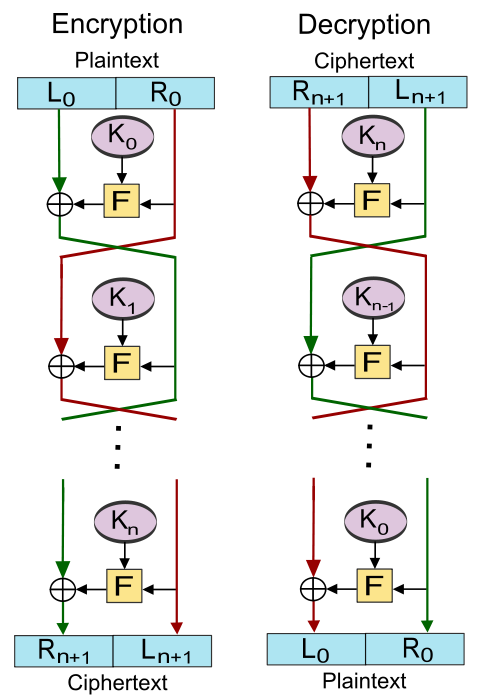• Non-Feistel ciphers
非费斯妥密码只能使用可逆组件。

## 2. Data Encryption Standard (DES)

### 2.1 简化版的 DES

• Key size = 10 bits (56 bits for DES)
• Number of rounds = 2 (16 for DES)
• Plaintext block = 8 bits (64 bits for DES)
• Ciphertext block = 8 bits (64 bits for DES)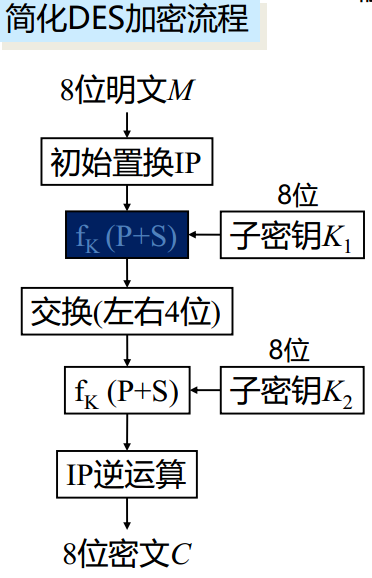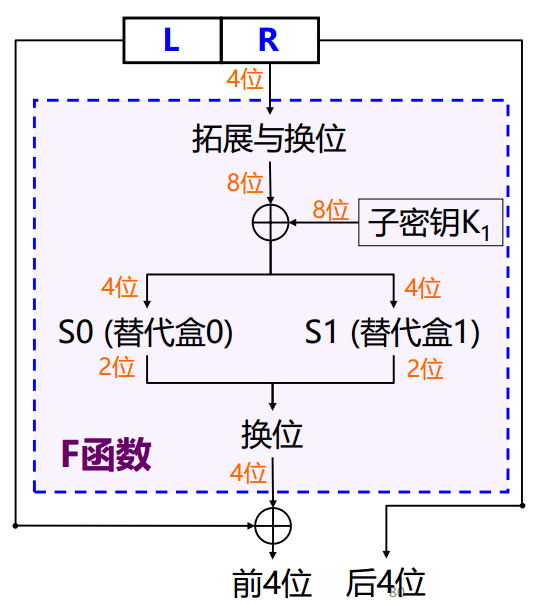### 2.2 DES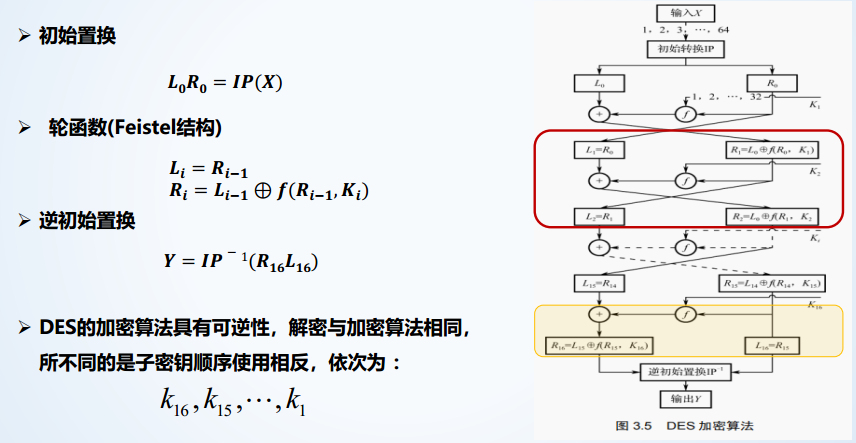• 初始置换 IP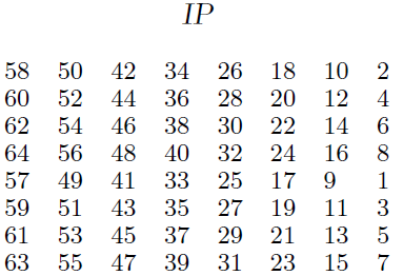• 轮函数

$\begin{array}{c} L_i = R_{i-1} \\ R_i = L_{i-1} \oplus F(R_{i-1},K_i) = L_{i-1} \oplus P(S(E(R_0) \oplus K_1)) \\ \end{array}$

• 初始置换 IP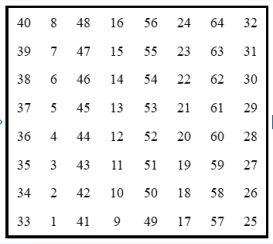• 轮密钥生成
置换 $PC_1$，循环左移，置换 $PC_2$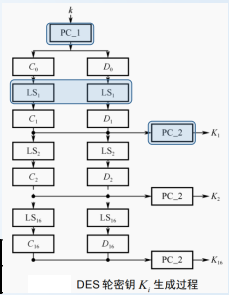### 2.3 DES 分析

• 雪崩效应：同一密钥加密仅一个 bit 位的不同明文，最后得到的密文大相径庭

• 完整性：一个密文 bit 位依赖与许多明文 bit 位

• 设计原则：

1. S 盒：提供混淆和扩散作用
2. P 盒：提供扩散作用
3. 轮函数：使用 16 轮 Feistel 加密